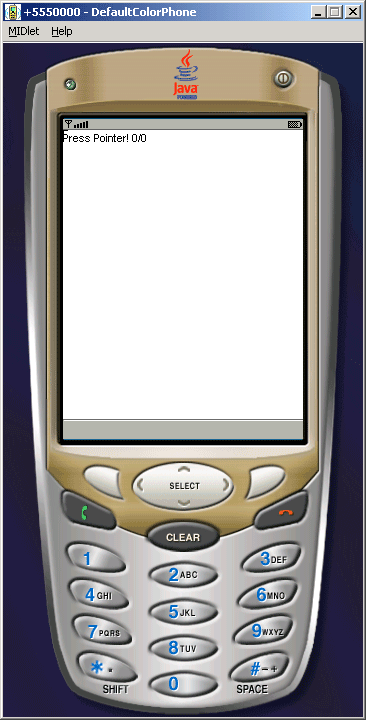Tutorials

# Pointer Example

This type of example is basically used in touch screen technology, here we are trying to create a pointer which location is based on the screen touch pointer. The source code for this small example of touch screen application.

This type of example is basically used in touch screen technology, here we are trying to create a pointer which location is based on the screen touch pointer. The source code for this small example of touch screen application.

# Pointer Example

This type of example is basically used in touch screen technology, here we are trying to create a pointer which location is based on the screen touch pointer. The source code for this small example of touch screen application.

 ``` public void pressPointer (int x, int y) {   action = "Pointer Pressed";   this.x = x;   this.y = y;   repaint ();   }```

is used to initialize the pointer location when pressed. The source code

 ```public void releasePointer (int x, int y) {   action = "Pointer Released";   this.x = x;   this.y = y;   repaint ();   }```

is used to released the pointer from his location. And the source code

 ```public void dragPointer (int x, int y) {   action = "Pointer Repeated";   this.x = x;   this.y = y;   repaint ();   }```

is used when repeating the pointer, then it relocate the location of the pointer. The Application is as follows:Source Code of PointerExample.java

 ``` import javax.microedition.midlet.*; import javax.microedition.lcdui.*; public class PointerExample extends MIDlet{   private Display display;   public void startApp(){   display = Display.getDisplay(this);   display.setCurrent (new PointerCanvas());    }   public void pauseApp(){}   public void destroyApp (boolean forced){} } class PointerCanvas extends Canvas {   String action = "Press Pointer!";   int x;   int y;   public void pressPointer (int x, int y) {   action = "Pointer Pressed";   this.x = x;   this.y = y;   repaint ();   }   public void releasePointer (int x, int y) {   action = "Pointer Released";   this.x = x;   this.y = y;   repaint ();   }      public void dragPointer (int x, int y) {   action = "Pointer Repeated";   this.x = x;   this.y = y;   repaint ();   }   public void paint (Graphics g) {   g.setGrayScale (255);   g.fillRect (0, 0, getWidth(), getHeight());   g.setGrayScale (0);   g.drawString (action + " " + x + "/" + y, 0, 0,    Graphics.TOP|Graphics.LEFT);   g.drawLine (x-4, y, x+4, y);   g.drawLine (x, y-4, x, y+4);   } }```